# 3.9 Energy barriers of individual grains

The requirement of large thermal stability of the recording media implies large energy barriers. Therefore, the next step is their evaluation for the grains of the previous section. For this purpose we used the Lagrange multiplier method presented in Section 2.3.6. Fig. 3.33 shows the energy barrier calculation for both model I and II as a function of the (perpendicular) interfacial exchange. Initially, the system is considered in the minimum that corresponds to all the moments aligned and to the coordinatesin the energy landscapes plotted in Figs. 3.34 and 3.35. Recall that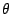is the average magnetization polar angle. The final state will be the equivalent minimum but with the moments pointing in the opposite direction, namely,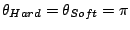. For small values of the interfacial exchange, there is an intermediate minimum (see Fig. 3.34) and, therefore, additional reversal modes and saddle points. In this situation the soft grain can switch first with very low energy barrier and the hard magnetic material will follow with a reduced energy barrier value. However, the energy barrier corresponding to the inverse process, namely, returning to the original minimum, is very small and the probability of the inverse process to take place is high. Fig. 3.34 shows the effective energy landscape and relevant configurations. The saddle points configurations are curled to minimize the magnetostatic energy of the soft material. For interfacial exchange energy higher than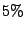of the bulk exchange in all cases, the energy barrier values saturate as a function of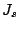and correspond to collective reversal. The collective modes are different for the two models. In model I the thermal mode is almost coherent (see Fig. 3.36(a)). The maximum energy barrier value is determined by the quantity. In the model II, the value of the energy barrier coincides with the domain wall energy in the hard magnetic material as observed by D.Suess [Suess 05b] and the saddle point is a domain wall centered in the hard material (see Fig. 3.36(b)). These differences can be appreciated in the effective energy Fig. 3.35. If the point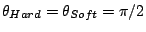is the saddle point, the energy barrier corresponds to coherent rotation. For a non-homogeneous mode the saddle point has to be elsewhere as in Fig. 3.35(b).Figure: Effective energy landscapes : (a) Model I,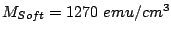and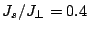(b) Model II,and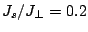.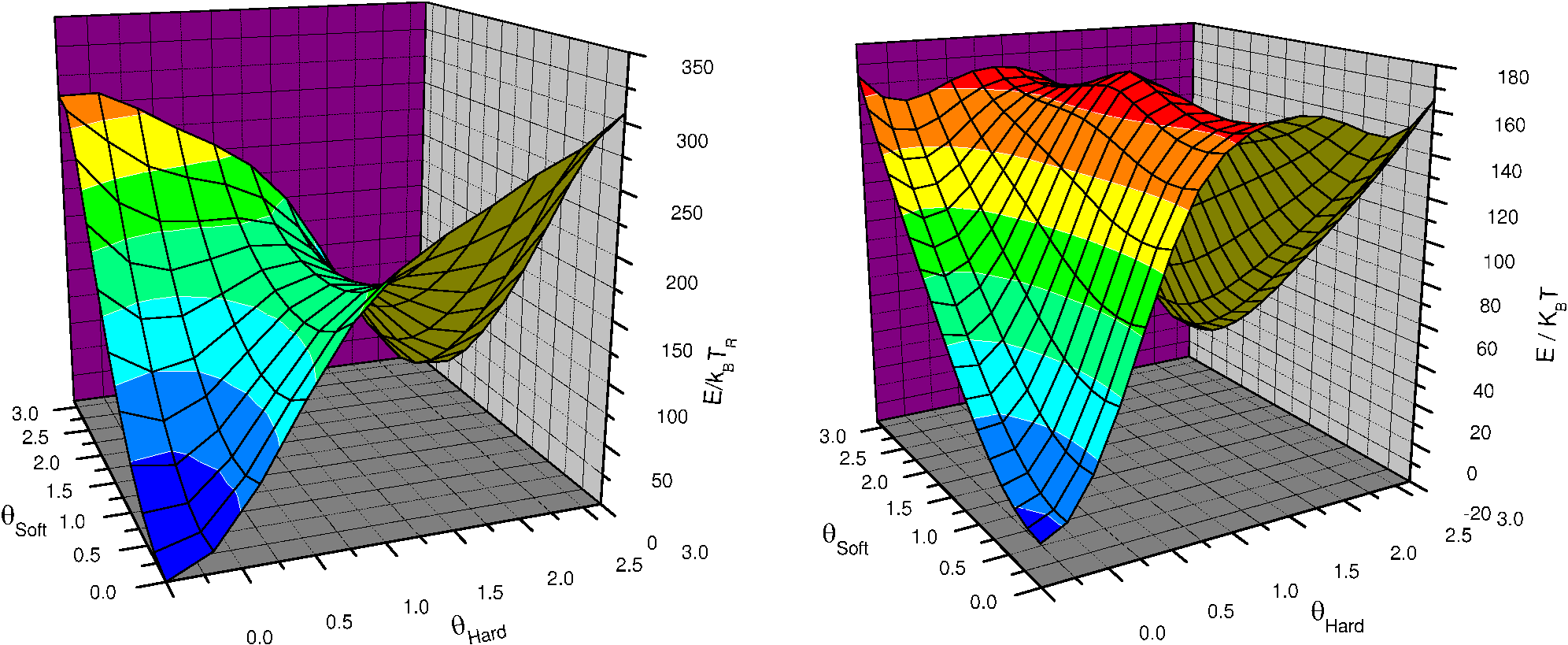(a) (b)
Figure 3.36: Saddle point configuration for different mechanism: (a) Coherent rotation (Model I) (b) Domain wall (Model II).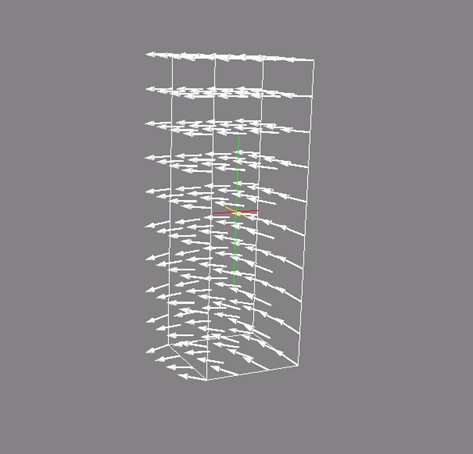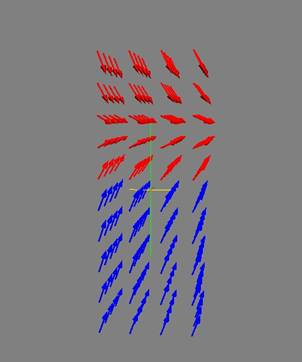(a) (b)

To get a better understanding of the energy barriers in a soft/hard material, we can examine the case of model I for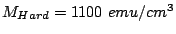,with a model similar to that of Ref. [Victora 05a]. The model supposes a macro-spin for each material. In such a model the energy is:(3.8)

where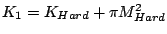and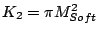are the effective anisotropy constants,is the number of atoms in the surface,is the volume of each material and finallyandare the unit vector of the macrospin of each grain. To analyze the problem, we have to consider the energy gradient and the Hessian matrix. For large values ofthere is only one energy barrier corresponding to the point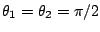which value is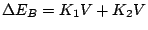. For small interfacial exchange, there are additional barriers and saddle points similar to the ones plotted in Fig. 3.34. The critical exchange for the crossover between the region is:(3.9)

In our system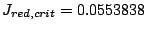. Finally, we can compare the analytical results with our simulations for the same values of the constants. From Fig. 3.37, it is clear that there is a good quantitative and qualitative agreement. These results indicate that the two macro-spins is a good approximation for small interfacial exchange. Nevertheless, this model is not valid for large exchange, where highly nonhomogeneous modes are expected.

Figure: (a) Comparison between the analytical model (solid lines) and numerical calculations (symbols) forandin model I. (b) Energy contour plot from Eq. (3.8) for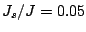.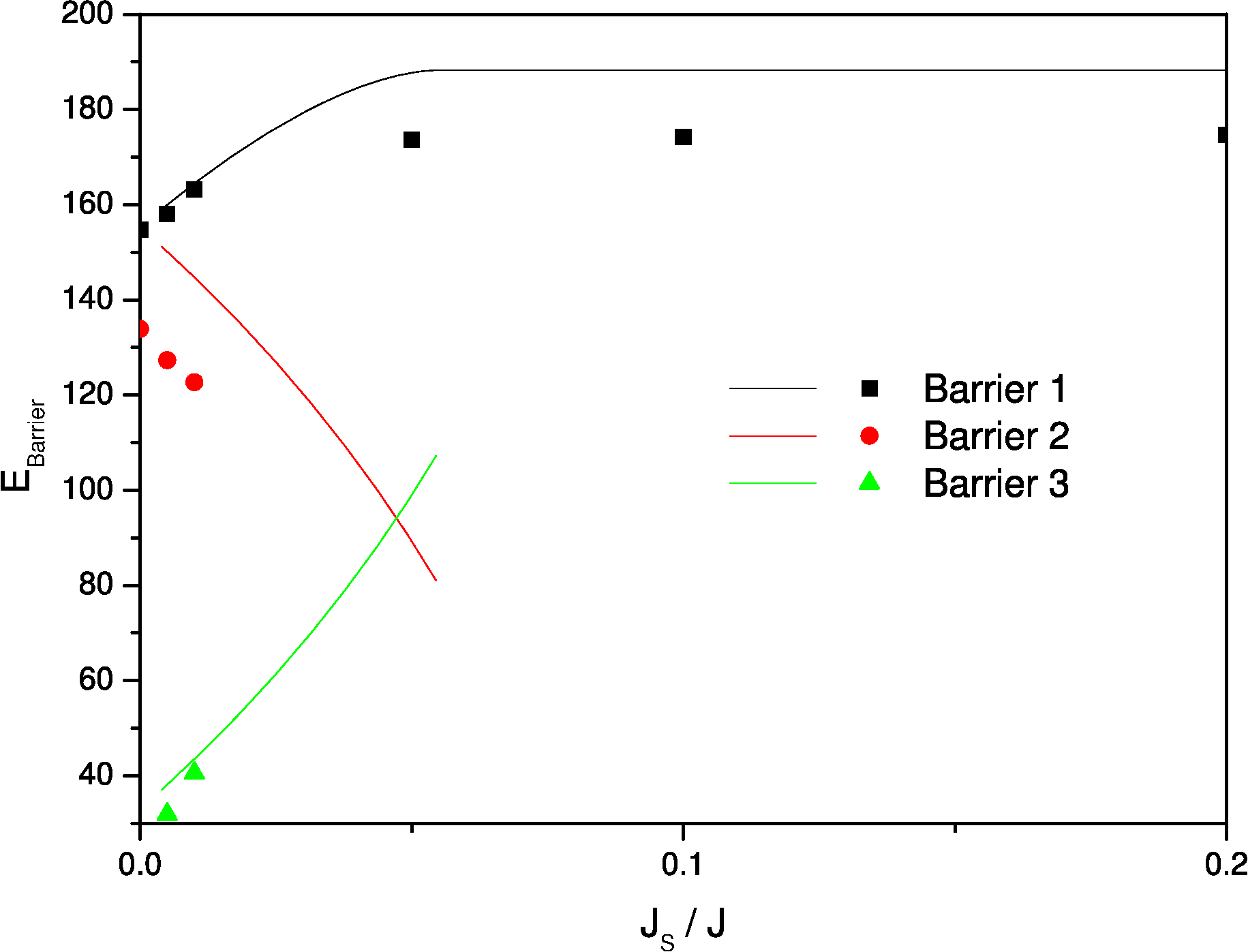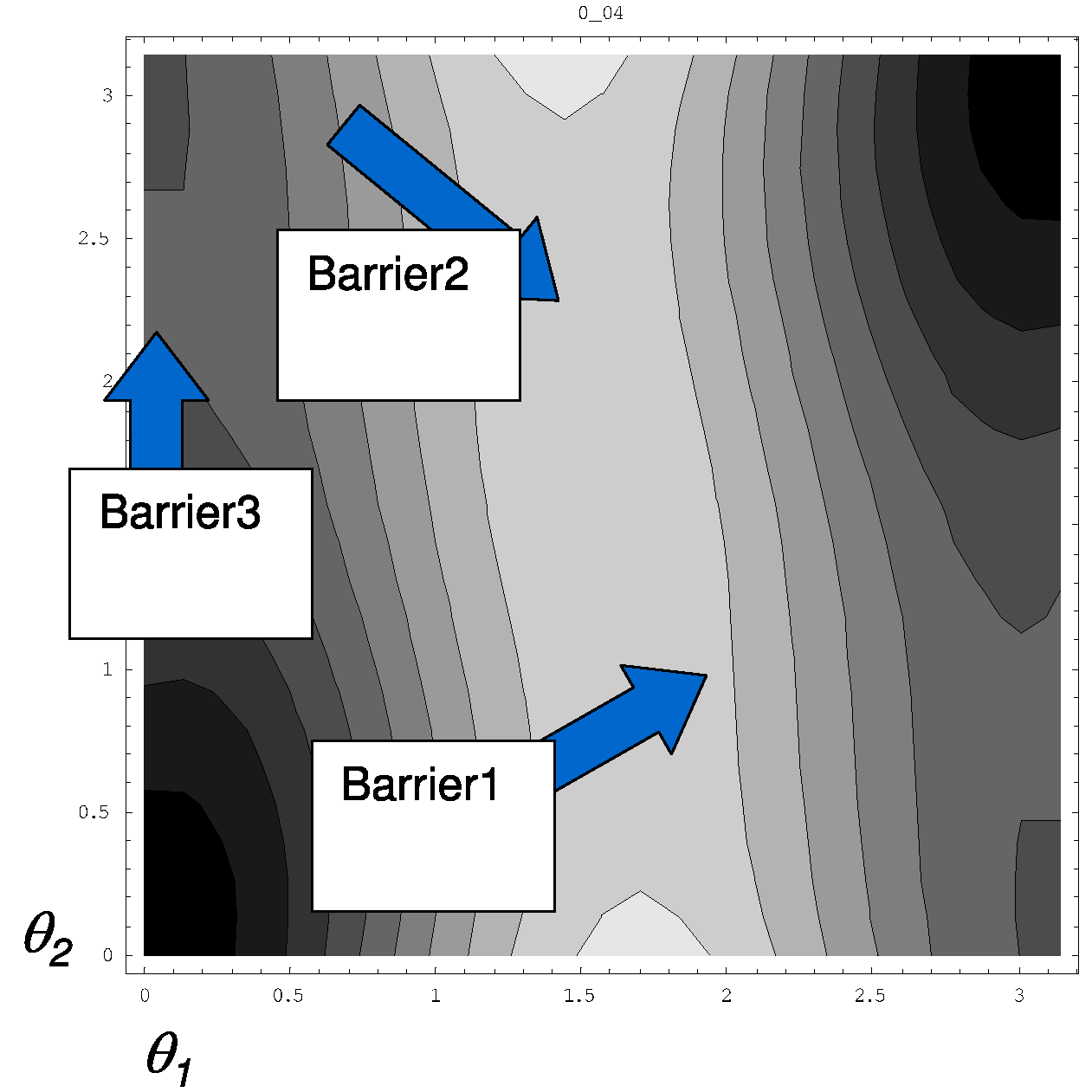(a) (b)
2008-04-04